# How to Write a Ratio as a Fraction

How to Write a Ratio as a Fraction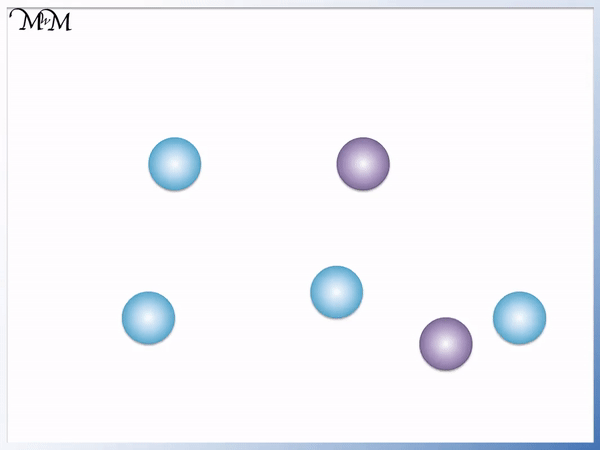• The sum of the parts in the ratio is the of our fractions.
• 4 + 2 = 6, so the denominators of both fractions will be 6.
• The separate parts in the ratio make the .
• On one side of the ratio we have 4 and the other side is 2, so our numerators are 4 and 2.
Each side of our ratio makes up the numerators on top.

Add the numbers in the ratio to get the denominators on the bottom. .• We have 5 blue counters to 6 purple counters.
• We write the counters in a ratio of 5:6.
• 5 + 6 = 11, so the denominator on the bottom of the fractions will be 11.
• We have 5:6, so we have 5 as one numerator and 6 as the other.
• 5:6 can be written as two fractions   5 / 11   and   6 / 11  .Supporting Lessons# Converting Ratios to Fractions

## How to Write a Ratio as Fractions

To write a ratio as fractions, add the total parts in the ratio to find the denominators and write each part of the ratio as the individual numerators.

When writing ratios as fractions, the number of fractions that are formed is the same as the number of parts in the ratio.

Here is a step-by-step guide to writing a ratio as a fraction:

1. Count the number of different terms in the ratio to find the number of fractions that will be made.
2. Add the different terms in the ratio to find the denominator that will be written on the bottom of all of the fractions.
3. Write each individual term of the ratio as the numerator for each of the separate fractions.

Here is an example of converting a ratio to two fractions.

In this example, there are 4 blue counters to 2 purple counters. The counters are in a ratio of 4:2.There are two terms in the ratio, 4 and 2. Therefore, we can write this ratio as two separate fractions: the fraction of counters that are blue and the fraction that are purple.

The first step in writing this ratio as a fraction is to add the two parts in the ratio to find the denominator, which is the number on the bottom of the fraction.

We add together the number of blue counters and the number of purple counters.

4 + 2 = 6

So, the denominator of each fraction is 6.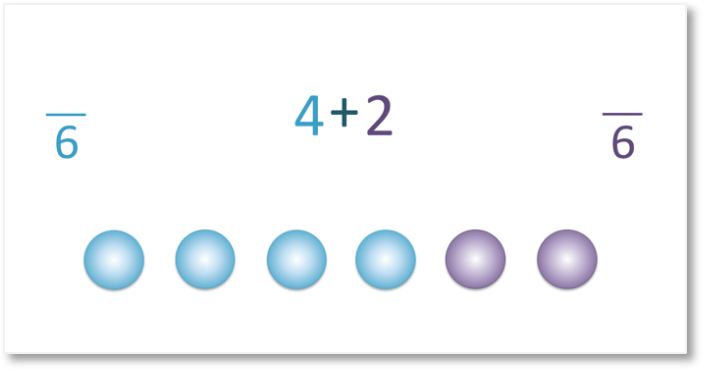The denominator tells us the total number of items in the ratio. In this example it tells us the total number of counters.

Next, we will find the fraction of the total counters that are blue.

According to the ratio 4:2, four of the counters are blue. This means that four out of six counters are blue. We take the 4 from the ratio and write it as the numerator on top of the fraction.

Therefore, the fraction of counters that are blue is   4 / 6.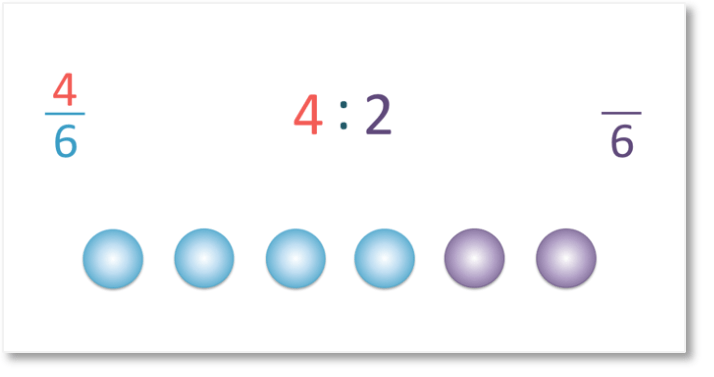Now, we’ll find the fraction of counters that are purple.

If we look at the ratio, we can see that two of the counters are purple. This means that two out of the six counters are purple. We take the 2 from the ratio and write it as the numerator of the fraction.

Therefore, the fraction of counters that are purple is   2 / 6.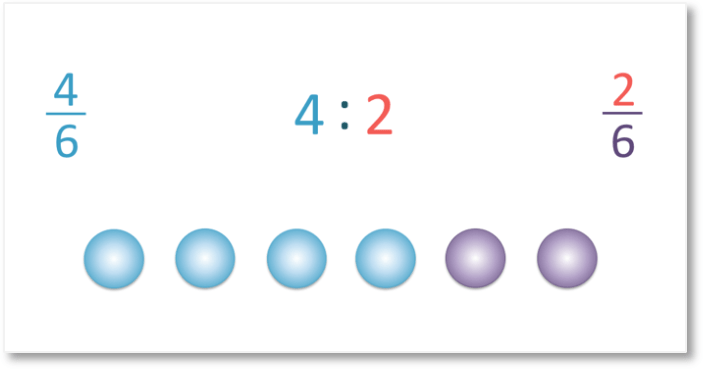The sum of the parts in the ratio tells us the denominator of the fractions.

Each part in the ratio tells us the numerators of the fractions.

To write a ratio as a fraction in its simplest form, add the parts of the ratio to form the denominator, write the individual parts of the ratio as the numerators and then divide the numerators and denominators of the fraction by the same amount if possible. Only divide the numerators and denominators if they can be divided exactly to produce a whole number.

The fractions that are formed from this ratio can be simplified by dividing both the numerators and denominators by 2.

The fraction   4 / 6   can be simplified to   2 / 3  and the fraction   2 / 6  can be simplified to   1 / 3 .

Here is another example of converting a ratio to a fraction.

We have boys and girls in a ratio of 5:8. We want to write the fraction of the total amount that are boys and the fraction of the total amount that are girls.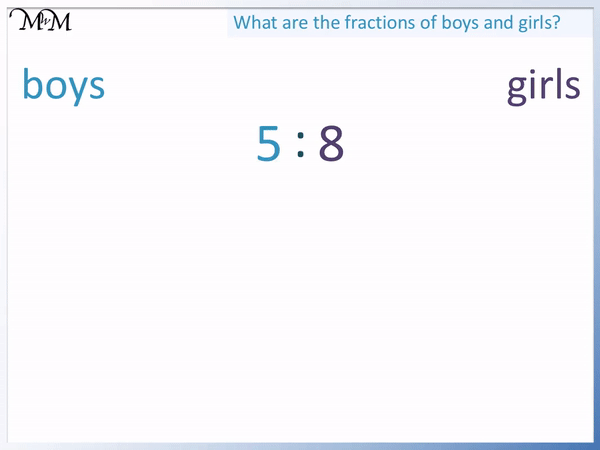We follow the steps as before.

5 + 8 = 13, so the denominators on the bottoms of the fractions will be 13.

We then put 5 and 8 as the numerators on the tops of the fractions.

5 / 13  of the people are boys and   8 / 13  of the people are girls.

We cannot simplify these fractions any further because 13 is a prime number and cannot be divided exactly by any smaller number.

Here is another example of writing a ratio as a fraction.

We have cat owners to dog owners in the ratio 12:7.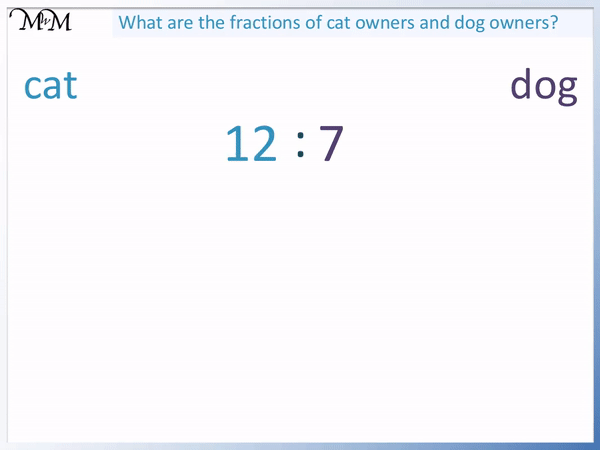The first step is to add the parts in the ratio together to find the denominators of the fractions.

12 + 7 = 19 and so, 19 is the denominator on the bottom of the fractions.

The next step is to write each separate part of the ratio as numerators on the tops of the fractions.

We have   12 / 19  and   7 / 19  .

This means that   12 / 19  are cat owners, while   7 / 19  are dog owners.

19 is a prime number and so it cannot be divided exactly by any number apart from 1 and itself. We cannot simplify these fractions any further.Now try our lesson on Writing Fractions and Ratios where we learn how to write and compare fractions and ratios for a given amount.error: Content is protected !!